### Home > CALC > Chapter 2 > Lesson 2.3.2 > Problem2-120

2-120.
1. For each part below, draw a graph of a function that meets the given conditions, if possible. If such a function is not possible, explain why. Homework Help ✎

1. g(x) is discontinuous at x = a, but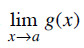exists.

2. g(x) is continuous at x = a, but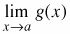does not exist.

3. g(x) is discontinuous at x = a, and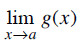does not exist.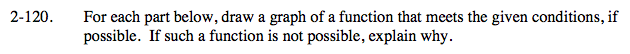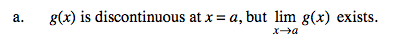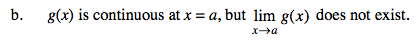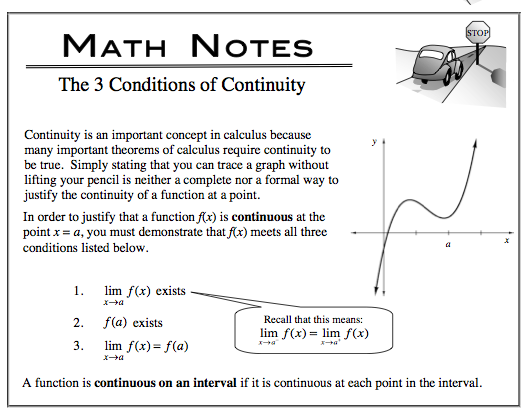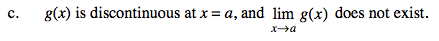Limits exist when both sides approach the same finite value. What does it mean for a limit to not exist?